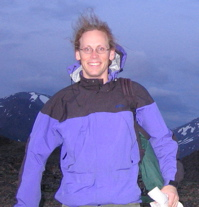# David Maxwell

My research has focused on:
• The construction of slices of spacetime in General Relativity.
• Inverse problems in glaciology.
These seem like bizarrely unrelated topics, but both apply partial differential equations at the boundary between mathematics and physics.

#### Slices of spacetime

• D. Maxwell, Conformal parameterizations of Kasner spacetimes, preprint, 2014

• D. Maxwell, The conformal method and the conformal-thin sandwich methods are the same, preprint, 2014 (arxiv:1402.5585)

• D. Maxwell, A model problem for conformal parameterizations of the Einstein constraint equations, Comm. Math. Phys., vol. 302, no. 3, pp. 697—736, 2011. (arxiv:0909.5674)

• D. Maxwell, A class of solutions of the vacuum Einstein constraint equations with freely specified mean curvature, Math. Res. Lett. 16 (2009), no. 4, 627 - 645. (arXiv:0804.0874)

• D. Maxwell, Rough solutions of the Einstein constraint equations, J. Reine Ang. Math., 590 (2006), 1 - 30 (gr-qc/0405088)

• D. Maxwell, Solutions of the Einstein constraint equations with apparent horizon boundaries, Comm. Math. Phys. 256 (2005), 561 - 583. (gr-qc/0307117)

• D. Maxwell, Rough solutions of the Einstein constraint equations on compact manifolds, J. Hyp. Diff. Eq. 2 (2005), 521 - 546. (gr-qc/0506085)

• J. Isenberg, D. Maxwell, D. Pollack, A gluing construction for non-vacuum solutions of the Einstein constraint equations, to appear Adv. Theor. Math. Phys., (2005). (gr-qc/0501083)

• D. Maxwell, Initial Data for Black Holes and Rough Spacetimes, Dissertation, University of Washington, 2004 (PDF)

#### Inverse problems in glaciology

• M. Habermann, M. Truffer, and D. Maxwell, Changing basal conditions during the speed-up of Jakobshavn Isbræ, Greenland, The Cryosphere, vol. 7, no. 6, pp. 1679—1692, 2013

• M. Habermann, D. Maxwell, and M. Truffer, Reconstruction of basal properties in ice sheets using iterative inverse methods, Journal of Glaciology, vol. 58, pp. 795—807, 2012

• D. Maxwell, Kozlov-Maz'ya iteration as a form of Landweber iteration, (to appear: Inverse Problems and Imaging)

• D. Maxwell, M. Truffer, and S. Avdonin, Inverse Methods for Reconstructing Basal Boundary Data Poster, AGU Fall Meeting, 2008.

• D. Maxwell, M. Truffer, S. Avdonin, and M. Steuffer, An iterative scheme for determining glacier velocities and stresses, J. Glac., 54 (2008), 888—898.

• S. Avdonin, V. Kozlov, D. Maxwell, and M. Truffer. Iterative methods for solving a nonlinear boundary inverse problem in glaciology, J. Inverse Ill-Posed Problems, 2008.

#### Non-Newtonian fluid flow

• D. Maxwell, A regularity technique for nonlinear Stokes-like elliptic systems, Navier-Stokes Equations and Related Nonlinear Problems (H. Amann, G.P. Galdi, K. Pileckas and V.A. Solonnikov eds.), VSP, 1998, pp. 165-181.

• D. Maxwell, On the regularity of a model non-Newtonian fluid, MSc Thesis, University of British Columbia, 1997 (PDF)